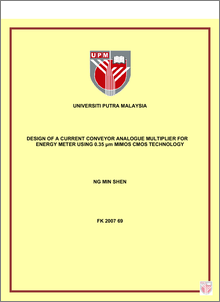# Design Of A Current Conveyor Analogue Multiplier For Energy Meter Using 0.35 Μm Mimos Cmos Technology

## Citation

Ng, Min Shen (2007) Design Of A Current Conveyor Analogue Multiplier For Energy Meter Using 0.35 Μm Mimos Cmos Technology. Masters thesis, Universiti Putra Malaysia.

## Abstract

Analogue VLSI circuits are essential in many real-time signal processing applications as naturally occurring signals are analogue. The four-quadrant analogue multiplier is a key building block in analogue signal processing circuits. It is used to construct circuits like the modulator and waveform generator. The ideal output (Vout) of a multiplier is related to the inputs by Vout = KmVXVY, where Km is the multiplier gain with units of V-1, and VX and VY are input voltages. In reality, imperfections exist in the multiplier gain, resulting in offsets and nonlinearities. Important parameters such as power dissipation, supply voltage, input dynamic range, bandwidth, total harmonic distortion (THD) and linearity are used to assess the performance of an analogue multiplier. Nowadays both digital and analogue systems are routinely integrated onto single chips. Digital circuits commonly use low-voltage supply and employ techniques to reduce power consumption. Mixed analogue-digital circuits must be designed to operate in a low-voltage, low-power environment. Conventional analogue multipliers designed with low supply voltage suffer from performance trade-offs, resulting in low bandwidth and low dynamic range because the design of analogue circuits is a trade-off of various performance parameters such as power dissipation, supply voltage, gain, linearity and noise. The objective of this research is to design a low-voltage, low-power CMOS analogue multiplier that will address the above problems. The multiplier is designed in a modified bridged-triode scheme (MBTS) and uses current conveyors. As all analogue circuits can be decomposed into several sub-circuits, the performance of these sub-circuits decides the characteristics of the resultant circuit structure. The proposed circuit makes use of the current conveyor’s many special features, such as high output impedance and large bandwidth, to construct a low-voltage fourquadrant multiplier. The analogue multiplier designed in this research operates with a supply voltage of ±1V. The total harmonic distortion obtained from this multiplier is less than two percent, the input operating swing is up to 1Vpp, and the bandwidth achieved is more than 100MHz. It is designed using a 0.35μm technology from the Malaysian Institute of Microelectronics (MIMOS). In addition, an RMS-to-DC converter is designed using the same low-voltage design technique used for designing the adaptively-biased low-voltage current mirror (ABLVCM). Then an energy meter is designed using this analogue multiplier and the RMS-to-DC converter.Preview
PDF
FK_2007_69.pdfView Item